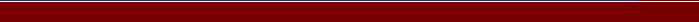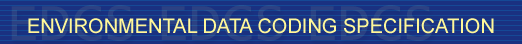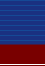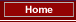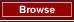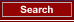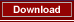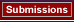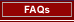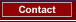## EA:  GEOPOTENTIAL_ALTITUDE

Status: STD 2005/06/07 423 The geopotential altitude H of a is its geopotential with respect to mean sea level (assumed at zero potential), expressed in geopotential metres, as shown in the equationH = (1/g0') · ∫0z g · dZwhere g0' is equal to 9,80665 m2/(s2 · gpm). REAL GEOPOTENTIAL_ENERGY_LENGTH IR [US_STDATM]